# Replace Piecewise-Constant Resistor with Switched Linear Components

This example shows how to convert a model that is nonlinear due to a Piecewise-Constant Resistor into a switched linear model making it compatible with Simscape™ to HDL Workflow.

### Introduction

Simscape™ to HDL Workflow supports conversion of Simscape switched linear models to functionally equivalent Simulink® models that are compatible for HDL code generation. In this example, you will learn how to replace a nonlinear element with equivalent switched linear components. Specifically, the model uses a Piecewise-Constant Resistor that contains events not supported by the Simscape HDL Workflow Advisor. You can convert the nonlinear Simscape™ model to a switched linear model and make it compatible for HDL code generation. For more information, see Generate HDL Code for Simscape Models.

To open the Simscape™ model. In the MATLAB® command prompt, enter:

```NonlinearModel = 'sschdlexVariableResistorExample'; open_system(NonlinearModel) set_param(NonlinearModel, 'SimulationCommand', 'update'); ```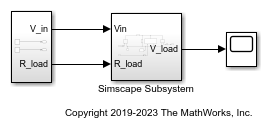To open the Simscape subsystem in the model, enter:

```open_system([NonlinearModel,'/Simscape Subsystem']) ```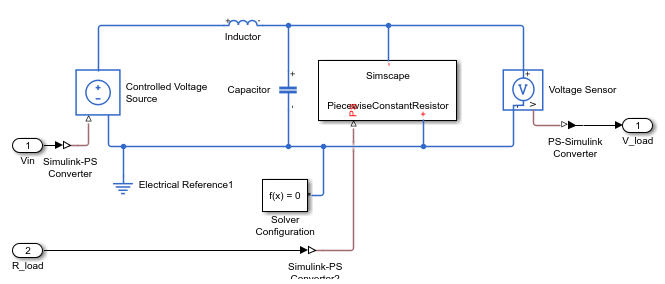This model is an RLC circuit with a Piecewise-Constant Resistor acting as a 'Load' resistance. For the Piecewise-Constant Resistor, the relationship between voltage `V` and current `I` is `V=I*R` where `R` is the numerical value presented at the physical signal port `R`.

To ensure a positive value for the resistance, any value below `1e-6` is replaced by `1e-6`. This resistor is Piecewise-Constant because the resistance only changes when the input value differs from the current resistance value by more than the set tolerance. Thus, a continuously changing input is converted to a discrete set of resistances.

In this model, the signal going into the Piecewise-Constant Resistor is a step function that changes from `2` to `3` at `t = 0.1` thus changing the load resistance from `2`Ω to `3`Ω.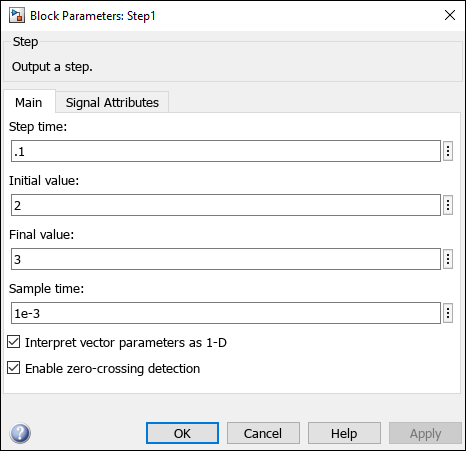To open the Simscape HDL Workflow Advisor, at the MATLAB® command-line, enter:

```sschdladvisor(NonlinearModel) ```

In the Simscape HDL Workflow Advisor, run the `Check model compatibility` task under `Code generation compatibility` subfolder. This task fails because of the presence of the Piecewise-Constant Resistor.### Replace Piecewise-Constant Resistor with switches and constant resistors

To convert this model to an equivalent switched linear model, replace the Piecewise-Constant Resistor with a set of switches and resistors for each desired value. To open the switched linear version of the model, in the MATLAB® command prompt, enter:

```SwitchedLinearModel = 'sschdlexVariableResistorSwitchedLinearExample'; open_system(SwitchedLinearModel) set_param(SwitchedLinearModel, 'SimulationCommand', 'update'); ```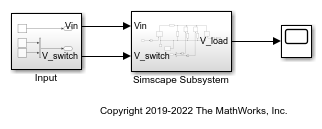The Piecewise-Constant Resistor is replaced by a resistor and a switch for each desired resistance. To recreate the behavior of a load resistance that changes from `2`Ω to `3`Ω at `t = 0.1` two resistors are used, one with a resistance of `2`Ω and the other with a resistance of `3`Ω. By closing and opening the switches the load resistance switches from `2`Ω to `3`Ω.

### Controlling the Switches

The switches must be turned on and off to provide the correct load resistance. To view the control signals for the switches, in the MATLAB® command prompt, enter:

```open_system([SwitchedLinearModel,'/Input']) ```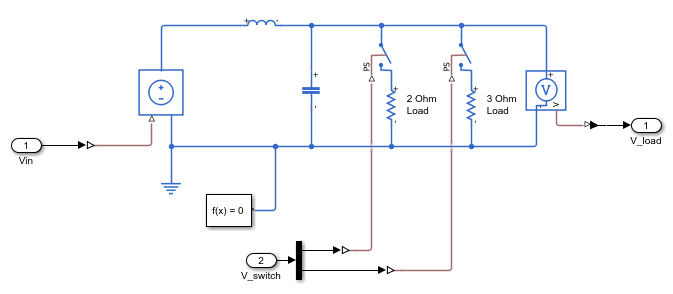To achieve the correct resistance, create two step functions:

1. To open the switch in series with the `2`Ω resistor at `t = 0.1`.

2. To close the switch in series with the `3`Ω resistor at the same time.

### Setup and Configuration

The default Solver Configuration settings for the switched linear model are:

• Solver type: `Backward Euler`

• Sample time: `1e-3`

In the model window, you can view the model statistics. Select the Debug tab and click Simscape > Statistics Viewer. This opens the Simscape Statistics window for `sschdlexVariableResistorExample` model.

### Generate HDL Implementation Model

For the switched linear components, open the Simscape HDL Workflow Advisor by running `sschdladvisor` function at the MATLAB® command prompt:

```sschdladvisor(SwitchedLinearModel) ```

To generate the HDL implementation model, in the `Implementation model generation` task drop-down list, right-click the `Generate implementation model` task, and then select the `Run to Selected Task` from the list.

After the task passes, you see a link to the HDL implementation model `gmStateSpaceHDL_sschdlexVariableResistorSwi`.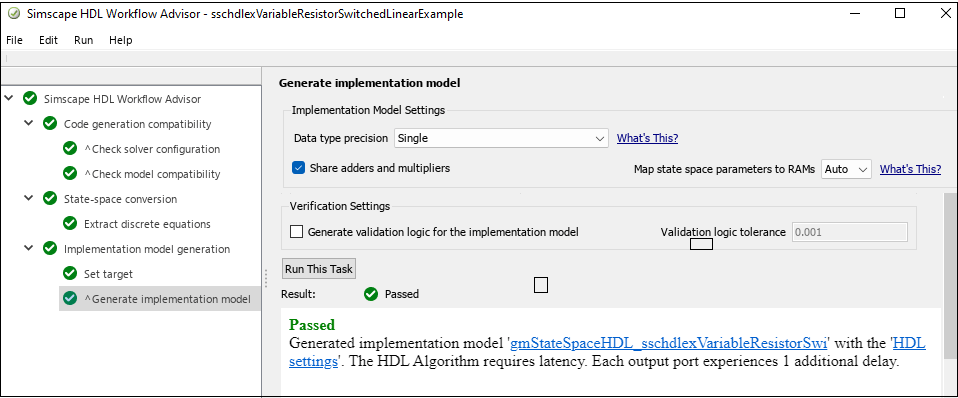Click on the link to open the generated implementation model.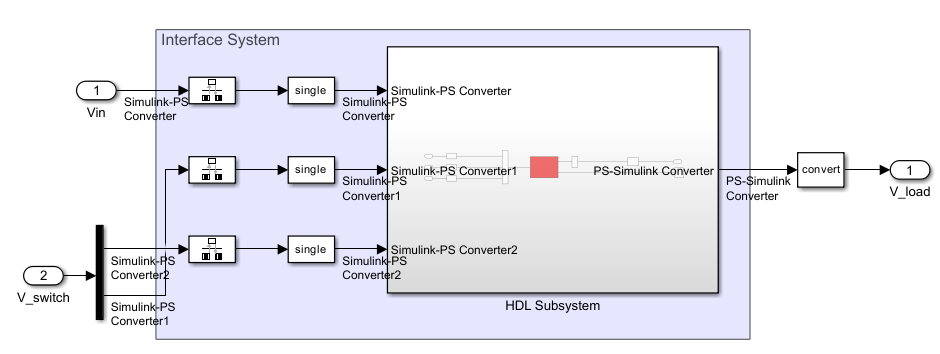When you run the Extract equations task, the task displays the number of states, input, output, modes, and differential variables for the Simscape network.When you run the Discretize equations task, the state-space representation is displayed in the right pane.### Generate HDL Code

To set up model parameters for HDL code generation, run `hdlsetup` function in MATLAB® command prompt:

```hdlsetup('gmStateSpaceHDL_sschdlexVariableResistorSwi') ```

Save the parameters for HDL implementation model.

```hdlsaveparams('gmStateSpaceHDL_sschdlexVariableResistorSwi') ```

Enable generation of the resource utilization report.

```hdlset_param('gmStateSpaceHDL_sschdlexVariableResistorSwi', 'ResourceReport', 'on') hdlset_param('gmStateSpaceHDL_sschdlexVariableResistorSwi', 'MaskParameterAsGeneric', 'off'); ```

Generate HDL code for the implementation model.

```makehdl('gmStateSpaceHDL_sschdlexVariableResistorSwi/Simscape Subsystem/HDL Subsystem'); ```

When you generate code, HDL Coder creates a code generation report. The resource utilization report in the High-level Resource Report indicates the amount of adders, multipliers, and registers that might be consumed on the target FPGA device.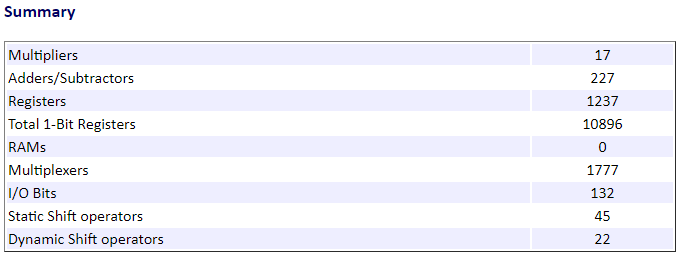By changing the Piecewise-Constant Resistor to a number of specified resistors that switch on and off, the model has been changed to a form that is compatible with the Simscape to HDL workflow.